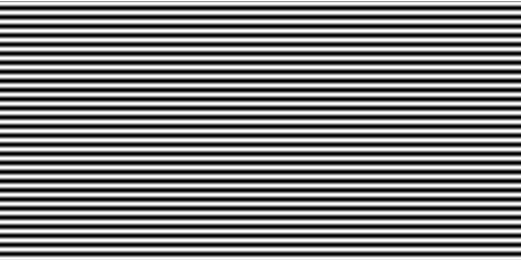# Building a raster in decimal degrees with areas in Sq.Km. as values

992
3
07-24-2019 01:18 AMNew Contributor II

I am trying to build a global raster at approx 500m resolution in geographic projection (cellsize 0.004167 decimal degrees), where values report the area of each cell in square meters.

I have built a Latitude grid that looks like this, where black is 0 and white is 90and I applied the formula, which didn't work:

111113 * ((0.004167 * Cos("LatitudeRaster_500m" * 0.017453292)) * 0.004167)

Where 111113 is the length of 1 degree of arc at the equator; LatitudeRaster_500m is the latitude grid reporting the value of latitude of each cell and 0.004167 is the cellsize)

I realized that the problem is with the Cos("LatitudeRaster_500m") that, if run as such, generate a strange raster where the sequence of between -1  and 1 occurs several times like this (while = 1; black = -1)while I was expecting a raster with a 1 in the center, gradually moving to a 0 where the latitude is 90 degrees.

Any suggestion?

Many thanks

Fabio

Tags (1)
3 RepliesbyMVP Legendary ContributorNew Contributor II

Thanks, but I don't want to project my raster layers. I have a pile of raster layers in geographic projection and would like to calculate areas using an additional raster with the area in Sq.Km of each cell as value.

FabioNew Contributor II

I solved the problem, the correct formula is:

(111319 * (0.004167 * Cos("Latitude_raster500" * 0.017453292)) * (0.004167 * 111319))

Where:

- 111319 is the length in meters of the arc of 1 degree at the Equator

- 0.004167 is the cell size in decimal degrees

- Latitude_raster500 is the raster layers of latitude values per cell

- 0.017453292 is the conversion factor from degrees to radiants (pi/180)

The output is a raster with the area of each cell as value

Best,

Fabio